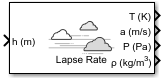Main Content

# Lapse Rate Model

Implement lapse rate model for atmosphere

• Library:
• Aerospace Blockset / Environment / Atmosphere

•## Description

The Lapse Rate Model block implements the mathematical representation of the lapse rate atmospheric equations for ambient temperature, pressure, density, and speed of sound for the input geopotential altitude. You can customize this atmospheric model by specifying atmospheric properties.

The ISA Atmosphere Model and Lapse Rate Model blocks are identical blocks. When configured for ISA Atmosphere Model, the block implements ISA values. When configured for Lapse Rate Model, the block implements the mathematical representation of lapse rate atmospheric equations.

The Lapse Rate Model block icon displays the input and output metric units.

## Limitations

• Below the geopotential altitude of 0 km and above the geopotential altitude of the tropopause, temperature and pressure values are held.

• Density and speed of sound are calculated using a perfect gas relationship.

## Ports

### Input

expand all

Geopotential height, specified as a scalar or array.

Data Types: `double`

### Output

expand all

Temperature, returned as a scalar or array, in K.

Data Types: `double`

Speed of sound, returned as a scalar or array, in m/s.

Data Types: `double`

Air pressure, returned as a scalar or array, in Pa.

Data Types: `double`

Air density, returned as scalar or array, in kg/m3.

Data Types: `double`

## Parameters

expand all

Customize various atmospheric parameters to be different from the lapse rate values. Selecting this check box converts the block from Lapse Rate Model to ISA Atmosphere Model.

#### Dependencies

Selecting this check box enables the parameters:

• Acceleration due to gravity (m/s^2)

• Ratio of specific heats

• Characteristic gas constant (J/Kg/K)

• Lapse rate (K/m)

• Height of troposphere (m)

• Height of tropopause (m)

• Air density at mean sea level (Kg/m^3)

• Ambient pressure at mean sea level (N/m^2)

• Lowest altitude (m)

#### Programmatic Use

 Block Parameter: `custom` Type: character vector Values: `'off'` | `'on'` Default: `'off'`

Acceleration from gravity (g). in m/s2, specified as double scalar.

#### Dependencies

This parameter is enabled when the Change atmospheric parameters check box is selected.

#### Programmatic Use

 Block Parameter: `g` Type: character vector Values: double scalar Default: `9.80665`

Ratio of specific heats γ, specified as a double value.

#### Dependencies

This parameter is enabled when the Change atmospheric parameters check box is selected.

#### Programmatic Use

 Block Parameter: `gamma` Type: character vector Values: double scalar Default: `1.4`

Characteristic gas constant (R), specified as double scalar, in J/Kg/K.

#### Dependencies

This parameter is enabled when the Change atmospheric parameters check box is selected.

#### Programmatic Use

 Block Parameter: `R` Type: character vector Values: double scalar Default: `287.0531`

Lapse rate of the troposphere, specified as double scalar, in K/m.

#### Dependencies

This parameter is enabled when the Change atmospheric parameters check box is selected.

#### Programmatic Use

 Block Parameter: `L` Type: character vector Values: double scalar Default: `0.0065`

Height of the troposphere (range of decreasing temperatures), specified as double scalar, in m.

#### Dependencies

This parameter is enabled when the Change atmospheric parameters check box is selected.

#### Programmatic Use

 Block Parameter: `h_trop` Type: character vector Values: double scalar Default: `11000`

Height of the tropopause (range of constant temperature), specified as double scalar, in m.

#### Dependencies

This parameter is enabled when the Change atmospheric parameters check box is selected.

#### Programmatic Use

 Block Parameter: `h_strat` Type: character vector Values: double scalar Default: `20000`

Air density at mean sea level, specified as double scalar, in Kg/m3.

#### Dependencies

This parameter is enabled when the Change atmospheric parameters check box is selected.

#### Programmatic Use

 Block Parameter: `rho0` Type: character vector Values: double scalar Default: `1.225`

Ambient pressure at mean sea level, specified as double scalar, in N/m2.

#### Dependencies

This parameter is enabled when the Change atmospheric parameters check box is selected.

#### Programmatic Use

 Block Parameter: `P0` Type: character vector Values: double scalar Default: `101325`

Ambient temperature at mean sea level (T0), specified as double scalar, in K.

#### Dependencies

This parameter is enabled when the Change atmospheric parameters check box is selected.

#### Programmatic Use

 Block Parameter: `K` Type: character vector Values: double scalar Default: `101325`

Lowest altitude above which temperature and pressure lapse, specified as double scalar, in m. Lowest altitude (m) must be below Height of tropopause.

#### Dependencies

This parameter is enabled when the Change atmospheric parameters check box is selected.

#### Programmatic Use

 Block Parameter: `h0` Type: character vector Values: double scalar Default: `0`

## Algorithms

These equations define the troposphere:

`$\begin{array}{c}T={T}_{0}-Lh\\ P={P}_{0}{\left(\frac{T}{{T}_{0}}\right)}^{\frac{g}{LR}}\\ \rho ={\rho }_{0}{\left(\frac{T}{{T}_{0}}\right)}^{\frac{g}{LR}-1}\\ a=\sqrt{\gamma RT}\end{array}$`

These equations define the tropopause (lower stratosphere):

`$\begin{array}{c}T={T}_{0}-Lhts\\ P={P}_{0}{\left(\frac{T}{{T}_{0}}\right)}^{\frac{g}{LR}}{e}^{\frac{g}{RT}}{}^{{}^{\left(hts-h\right)}}\\ \rho ={\rho }_{0}{\left(\frac{T}{{T}_{0}}\right)}^{\frac{g}{LR}-1}{e}^{\frac{g}{RT}}{}^{{}^{\left(hts-h\right)}}\\ a=\sqrt{\gamma RT}\end{array}$`

where:

 T 0 Absolute temperature at mean sea level in kelvin (K) ρ 0 Air density at mean sea level in kg/m 3 P 0 Static pressure at mean sea level in N/m 2 h Altitude in m hts Height of the troposphere in m T Absolute temperature at altitude h in kelvin (K) ρ Air density at altitude h in kg/m 3 P Static pressure at altitude h in N/m 2 a Speed of sound at altitude h in m/s 2 L Lapse rate in K/m R Characteristic gas constant J/kg-K γ Specific heat ratio g Acceleration due to gravity in m/s 2

 U.S. Standard Atmosphere., Washington, D.C.: U.S. Government Printing Office, 1976.

## SupportGet trial now